## Prove a consequence first

### Quick description

If one wants to show "If A, then B", first find an interesting consequence C of B that looks easier to prove than B itself, but not so simple that it can be immediately deduced from A. Then try to prove "If A, then C". Finally, show "If A and C, then B". The point is that this factors the original problem into two simpler ones. If one believes that the original implication is true, then the two sub-implications must be true also, so one is not "losing" anything by trying this method.

A variant approach, once one has successfully obtained "If A, then C", is to deconstruct that proof in order to find out what made it work; this can then give valuable clues as to how to then prove the stronger statement "If A, then B". This tends to work well when C acts as a "simplified toy model" of B.

### Example 1

For instance, if trying to prove an identity of the form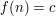, where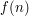is some expression depending on a parameter, andis independent of, one might first try to establish the simpler statement "is independent of", and then to establish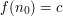for some special value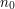of(note thatcould be an asymptotic value, such as infinity).

Note that many proofs of identities by induction are basically of this form.

### Independent

Proving the consequence first is a great way to simplify a problem.
But I don't understand the example. What exactly does "independent" mean. How can f(n) be independent of n?

Sorry if this is a stupid question, but I would really like to understand this.

### is independent of if theis independent ofif the value ofdoes not actually depend on, i.e. it is constant as a function of. For instanceis independent of, as it is always.

Equivalently,is independent ofif one has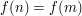for all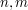.

### Thank you

That's a great explanation. Thanks.

## Post new comment

(Note: commenting is not possible on this snapshot.)

Before posting from this form, please consider whether it would be more appropriate to make an inline comment using the Turn commenting on link near the bottom of the window. (Simply click the link, move the cursor over the article, and click on the piece of text on which you want to comment.)